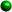Homework Tips for Solving Systems of EquationsSolving Systems of Equations by AddingLook at both equations and notice whether or not the sum of one of the variables is "0" when added. If so, add the two equations together and solve for one of the variables. Substitute back in one of the equations to solve for the other variable.If the sum of one of the variables does not equal "0" , then we make the sum of one of the variables equal "0". We can do this by multiplying one of the equations by -1 if one of the variables has the same coefficient in both equations.If we do not have one set of matching coefficients in the two equations, then we make this happen by multiplying one or both of the equations by numbers that will make the sum of one of the variables equal "O". Solving Systems of Equations by SubstitutionOne of the equations is already solved for one of the variables in terms of the other. Substitute this part of the equation into the other equation.